Next: Geodesics Up: The Theory of General Previous: The basic concepts

# The Kerr metric

The Schwarzschild metric describes space-time around a spherical mass M which does not have any angular momentum J. The Schwarzschild metric is:where the lapse function / gravitational redshift factor is: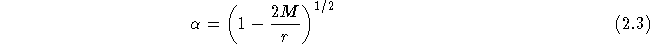The more general Kerr metric describes space-time around a spherical mass M which does have an angular momentum J, such as a rotating black hole. The Kerr metric is:where the Boyer-Lindquist coordinates are: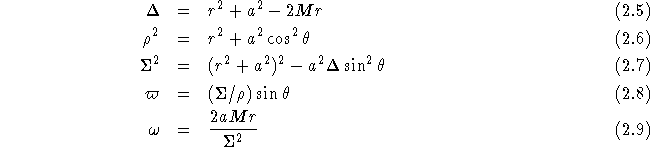and where the rotation parameter a is defined by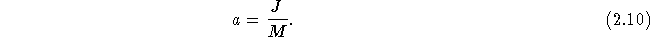One has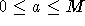; an object with higher angular momentum than corresponding to a = M cannot stick together.

The horizon is the surface which one when using the coordinates of a distant observer in flat space will never see anything pass. It is not a real singularity, but merely a coordinate singularity. It is given by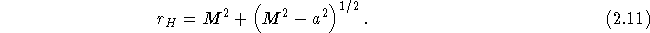Another interesting surface is that given by the static limit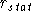, within which is is compulsory to move along the direction of rotation, since space-time is rotating with so high speed, that even when one is moving with the speed of light in the opposite direction, one will still be moving in the direction of rotation.is given by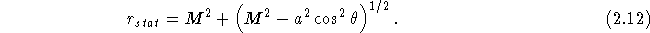The area within the static limit but outside the horizon is called the ergosphere.

Note, that we in all of the above have used ``natural units'', viz. c = G = 1. It might, however, be useful to know what absolute sizes I will be talking about when I say that r is 2, or M is 1. This can be derived by writing the equation for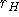with c and G included: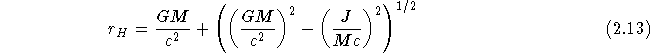(Berry 1976). We see that length over mass is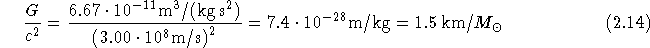Web Exhibition: Null Geodesics Around a Kerr Black HoleBo Milvang-Jensen (milvang@astro.ku.dk)
Mon Jun 17 11:54:08 MDT 1996